# CBSE Class 10 Maths Chapter 3 - Pair of Linear Equations in Two Variables Objective Questions

Chapter 3- Pair of Linear Equations in Two Variables from CBSE Class 10 Maths textbook explains all about the pair of linear equations in two variables. Two linear equations in two variables also called the pair of linear equations in two variables can be represented graphically and algebraically. Algebraically, students can use the Substitution method, Elimination method or Cross-multiplication method to solve the pair of linear equations in two variables.

From this chapter, we have compiled some 20 MCQs, which have been categorized topic-wise in the PDF link given below:

### List of Sub-Topics Covered

Some of the main sub-topics covered in this chapter of the CBSE Class 10 Maths have been listed below. Students also find these CBSE Class 10 Maths Objective Questions very useful. Students can practice the MCQs from the chapter given topic wise and master the concepts from the topics of this chapter.  Here are the list of topics given:

3.1 Algebraic Solution (4 MCQs Listed From This Topic)

3.2 All about Lines (4 MCQs From The Topic)

3.3 Basics Revisited (4 MCQs From This Topic)

3.4 Graphical Solution (4 MCQs Listed From The Topic)

3.5 Solving Linear Equations (4 MCQs From The Mentioned Topic)

## Download Free CBSE Class 10 Maths Chapter 3- Pair of Linear Equations in Two Variables Objective Questions PDF

### Algebraic Solution

1. Half the perimeter of a rectangular room is 46 m, and its length is 6 m more than its breadth. What is the length and breadth of the room?
1. 2m, 20m
2. 2m, 3m
3. 56m, 40m
4. 26m, 20m

Solution: Let l and b be the length and breadth of the room. Then, the perimeter of the room = 2(l+b) metres

From question, l=6+b… (1)

×2(l+b) =46⟹l+b=46… (2)

Using Substitution method:

Substituting the value of l from (1) in (2), we get

6+b+b=46

⇒ 6+2b=46⟹2b=40

⟹b=20 m.

Thus, l=26 m

1. Solve the following pair of equations:

2x+y=7

3x+2y=12

Choose the correct answer from the given options.

1. (-3,2)
2. (1,0)
3. (3,2)
4. (2,3)

Solution: We have,

2x+y=7    … (1)

3x+2y=12… (2)

Multiply equation (1) by 2, we get:

2(2x+y) =2(7)

⇒4x+2y=14… (3)

Subtracting (2) from (3) we get,

x=2

Substituting the value of x in (1) we get,

2(2) +y=7⟹y=3

Thus, the solution for the given pair of linear equations is (2, 3).

1. Solve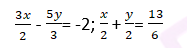1. Y= 51/19
2. X= 51/19
3. Y= 94/57
4. X= 117/54

Solution: (3x/2) – (5y/3) =2

LCM of 2 and 3 is 6. Multiply by 6 on both sides

9x−10y=−12 ————- (1)

(X/2) + (y/2) = 13/6

LCM is 6. Multiply by 6 on both sides

3x+3y=13 ————–(2)

Multiply equation (2) by 3 to eliminate x; so we get,

9x+9y=39……………(3)

Subtract (3) from (1) we have

−19y=−51⇒y= 51/19

Substitute this in one of the equation and we get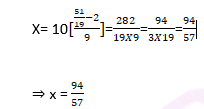1. Given: 3x–5y=4; 9x=2y+7

Solve above equations by Elimination method and find the value of x.

1. X= 9/13
2. Y= 5/13
3. X= (-5) / 13
4. Y= 9/13

Solution: Given:

3x–5y=4……(1)

9x=2y+7

9x−2y=7…..(2)

Multiply equation (1) by 3

⇒9x–15y=12…….(3)

Subtracting (2) from (3) we get,

−13y=5

Y= – ( (-5) / 13)

Substituting the value of y in (2)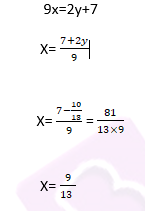1. Choose the pair of equations which satisfy the point (1,-1)
1. 4x–y=3,4x+y=3
2. 4x+y=3,3x+2y=1
3. 2x+3y=5,2x+3y=−1
4. 2x+y=3,2x–y=1]

Solution: For a pair of equations to satisfy a point, the point should be the unique solution of them.

Solve the pair equations 4x+y=3,3x+2y=1

let 4x+y=3…..(1)

and 3x+2y=1 …..(2)

y=3−4x   [ From (1)]

Substituting value if y in (2)

3x+2y=1

3x+2(3−4x)=1

3x+6−8x=1

−5x=−5⇒x=1

Substituting x = 1 in (1),

4(1)+y=3⇒y=−1

⇒ (1,-1) is the solution of pair of equation.

∴ Pair of equations which satisfy the point (1,-1)

Note: – We can also substitute the value (1,-1) in the given equations and check if it satisfies the pair of equations or not. In this case it only satisfies the pair of equation 4x+y=3, 3x+2y=1 and hence (1, -1) is the unique solution of the equation.

1. 54 is divided into two parts such that sum of 10 times the first part and 22 times the second part is 780. What is the bigger part?
1. 34
2. 32
3. 30
4. 24

Solution: Let the 2 parts of 54 be x and y

x+y = 54…. (i)

And 10x + 22y = 780 ——————– (ii)

Multiply (i) by 10, we get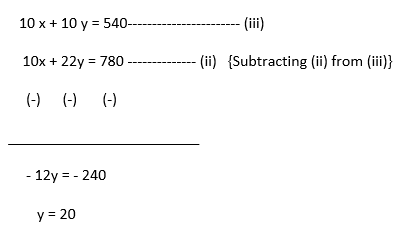Substituting y = 20 in x + y = 54, we have x + 20 = 54; x = 34

Hence, x = 34 and y = 20.

1. What are the values of ab and c for the equation y=0.5x+√7 when written in the standard form: ax+by+c=0?
1. 0.5, 1, √7
2. 0.5, 1, – √7
3. 0.5, -1, √7
4. -0.5, 1, √7

Solution: Y= 0.5X + √7

⇒0.5x−y+ √7= 0

The general form of an equation is ax+by+c=0.

Here, on comparing, we get

a=0.5, b=−1 and c= √7

1. Which of the following pair of linear equations has infinite solutions?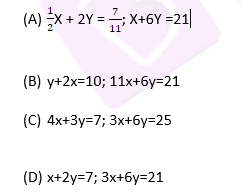Solution: If two equations are consistent and overlapping, then they will have infinite solutions. Option A consists of two equations where the second equation can be reduced to an equation which is same as the first equation.

x+2y=7…. (i)

3x+6y=21….. (ii)

Dividing equation (ii) by 3, we get

x+2y=7 which is the same as equation (i).

The equations coincide and will have an infinite solution.

Alternate Method:

Let the two equations be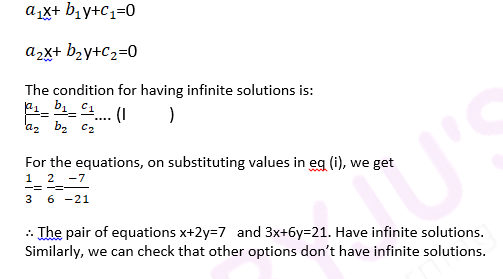### Basics Revisited

1. Which of these points lie on the line 7x+8y=61
1. (3,4)
2. (2,5)
3. (-3,7)
4. (3,5)

Solution: Substituting the value of x = 3 and y = 4,

7x+8y=61 = 7(3) +8(4) =53.

Substituting the value of x = 2 and y = 5,

7x+8y=61 = 7(2) +8(5) =54.

Substituting the value of x = -3 and y = 7,

7x+8y=61 = 7(−3) +8(7) =35.

Substituting the value of x = 3 and y = 5,

7x+8y=61 = 7(3) +8(5) =61

Hence, (3, 5) lies on the given line

1. Which of these following equations have x=−3, y=2 as solutions?
1. 3x−2y=0
2. 3x+2y=0
3. 2x+3y=0
4. 2x−3y=0

Solution: Substituting the values in LHS,

L.H.S=2x+3y

L.H.S=2(−3) +3(2)

L.H.S=0=R.H.S

Hence x=−3, y=2 is the solution of the equation 2x+3y=0

1. If y = 1/2 (3x+7) is rewritten in the form ax+by+c=0, what are the values of a, b and c?
1. ½, 7/2, 3/2
2. 7,2,3
3. -2, 3, -7
4. -3,2,-7

Solution: The given equation is:

y = 1/2 (3x+7)

Simplifying the equation we get:

2y−3x−7=0

⇒−3x+2y−7=0   (1)

Thus, the value of a, b and c is -3, 2 and -7 respectively.

The equation can also be written as,

3x−2y+7=0

Thus, the value of a, b and c is +3, -2 and +7 respectively.

The option -3, 2 and -7 is correct [Since +3, -2 and +7 is not an option]

1. x−y=0 is a line:
1. Passing through origin
2. Passing through (1,-1)
3. || to y axis
4. || to x axis

Solution: x−y=0, is a line passing through the origin as point (0, 0) satisfies the given equation

### Graphical Solution

1.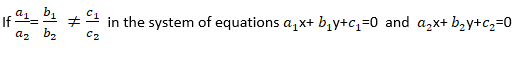Statement 1: This is the condition for inconsistent equations

Statement 2: There exists infinitely many solutions

Statement 3: The equations satisfying the condition are parallel

Which of the above statements are true?

1. S1 only
2. S1 and S2
3. S1 and S3
4. S2 only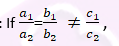The condition is for inconsistent pair of equations which are parallel and have no solution.

∴ Statement 1 and 3 are correct

1.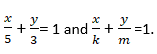Choose the correct statement.

1. For k3(m/5), a unique solution exists
2. For k=3 (m/5), infinitely many solutions exists
3. For k=  5( m/3), a unique solution exists
4. Fork= 5(m/3) , infinitely many solutions exist

Answer: For k= 5 (m/3), infinitely many solutions exist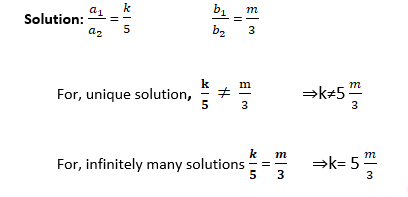1. The figure shows the graphical representation of a pair of linear equations. On the basis of graphthe pair of linear equation gives _______________solutions.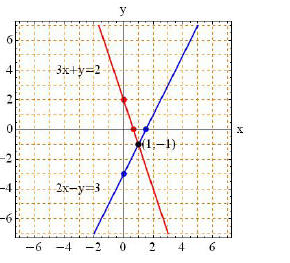1. Four
2. Only one
3. Infinite
4. Zero

Solution: If the graph of linear equations represented by the lines intersects at a point, this point gives the unique solution. Here the lines meet at the point (1,-1) which is the unique solution of the given pair of linear equations.

1. For what value of k, the pair of linear equations 3x+ky =9 and 6x+4y=18 has infinitely many solutions?
1. -5
2. 6
3. 1
4. 2

Solution: Given equations gives infinitely many solutions if,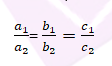The given linear equations are:

3x+ky=9; 6x+4y=18.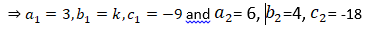⇒ 3/6 = k/4 = (-9)/ (-18)

⇒ ½ = k/4

⇒ k = 2

### Solving Linear Equations

1. Solve the following pair of linear equation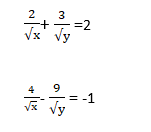1. 9,8
2. 4,9
3. 3,2
4. ½ , 1/3

Solution: The pair of equations is not linear. We will substitute 1/x as u2 and 1/y as v2 then we will get the equation as

2u+3v=2

4u−9v=−1

We will use method of elimination to solve the equation.

Multiply the first equation by 3, we get

6u+9v=6

4u−9v=−1

10u=5

u= ½

Substituting u in equation 4u−9v=−1 we get v= 1/3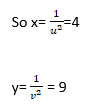1. Solve the following pair of equation

(7x-2y) / xy=5

(8x+6y) /xy =15

1. None of these
2. 2, not defined
3. 5/2 , not defined
4. (-2) /5 , not defined

Answer: (D) (-2) /5 , not defined

Solution: First separate the variables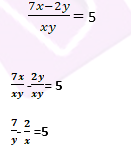Similarly we can do separation of variables for second equation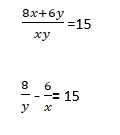Now we see that the equation is not linear.

So we will substitute 1/x = u and 1/y = v

The pair of equation can be written as

-2u+7v=5

-6u+8v=15

We can solve the pair of equation by method of elimination.

-6u+21v=15—————————- (1)

-6u+8v = 15—————————- (2)

Subtracting (2) from (1), we get 13v = 0; v = 0

Substituting v = 0 in -2u + 7v = 5

we get u= – (5/2)

1/x= u and 1/y = v

∴x = − (2/5) and y = not defined

1.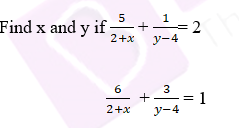1. x = -2,  y = 2
2. x = 7, y = -8
3. x = 0, y = 8
4. x = 1, y = 7

Answer: (D) x = 1, y = 7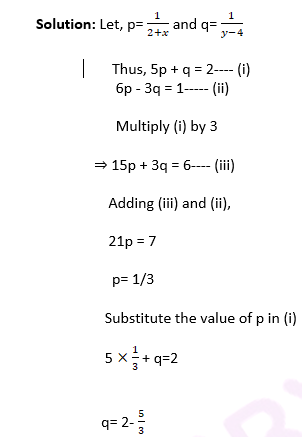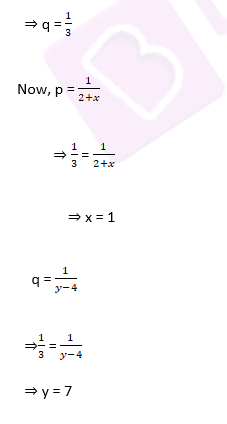1. Solve the following pair of equations:

(1/x) + (3/y) =1

(6/x)- 12/y=2

1. X= 5/3 , Y= 15/2
2. x=4,y=9
3. x=3,y=11
4. X= ¾ , Y= 7/3

Answer: (A) X= 5/3 , Y= 15/2

Solution: Let 1/x= a and 1/y = b

(As x≠0, y≠0)

Then, the given equations become

a+3b=1 … (1)

6a−12b=2 … (2)

Multiplying equation (1) by 4, we get 4a+12b=4 … (3)

On adding equation (2) and equation (3), we get 10a=6

⇒a= 3/5

Putting a= 3/5 in equation (1), we get

(3/5) + 3b =1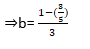⇒b= 2/15

Hence, x= 5/3 and y= 15/2

The students appearing for the board examinations will find it helpful in scoring well, as according to the latest modified exam pattern, the question paper will contain more objective type questions.

Keep visiting BYJU’s to access free study materials and exam preparation tools for all subjects.